# Construct a triangle ABC in which BC= 5 cm, AB- AC= 2.8 cm and $\angle B = {40^ \circ }$ .Verified
182.4k+ views
Hint: A triangle is a three sided quadrilateral and to construct a triangle one needs to draw a line segment and suppose it to be the base of the triangle. To construct a triangle one needs a protractor or a compass, a ruled scale.

Since we have been given the length of a line segment BC as 5 cm. let us draw a line segment BC using the ruler and suppose it to be the base of the triangle.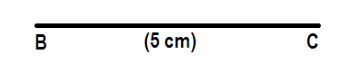We have the measure of angle B, therefore using the protractor we will make the $\angle PBC$ of measure equal to the $\angle B = {40^ \circ }$ and extend the ray .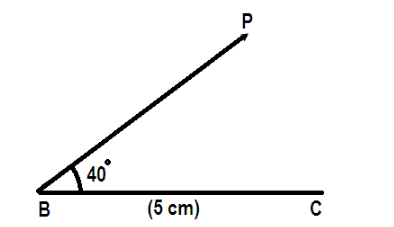Now from the ray AP cut off the line segment BD equal to ‘l’ which is the given difference of AB- AC = 2.8 cm. Stretch your compass 2.8 cm and put the needle of the compass at B cut off BD from the ray AP.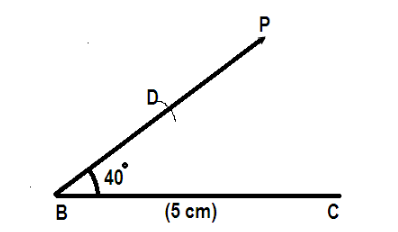Now join C and D. Construct the perpendicular bisector of CD meeting BP at C where the perpendicular bisector is drawn by drawing an arc on both the sides of DP with the help of compass taking C and D as centres.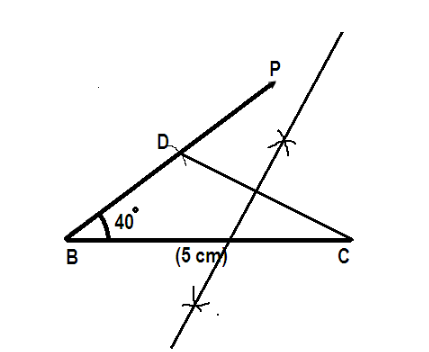Now extend the ray BP such that it meets the perpendicular bisector at the point A and then join A and C to draw the complete triangle ABC.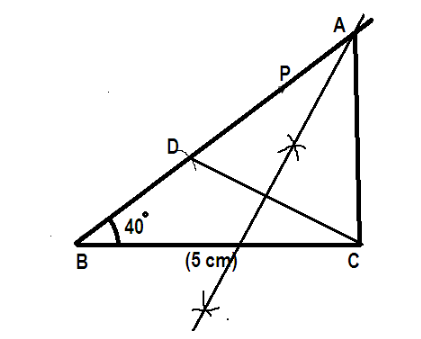Hence this is the triangle constructed.

Note: Carefully make the perpendicular bisector of CD with the help of the compass, perpendicular bisector is basically the line which bisects the given line segment and is perpendicular to that particular line segment.